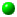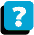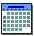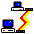Module 5.1 Notes "Simple, Joint, Marginal and Conditional Probabilities"

 Index to Module 5 Notes5.1: Simple, Joint, Marginal and Conditional Probabilities5.2: Confidence Interval and Hypothesis Testing for a Proportion5.3: Multiple Sample Tests with Categorical Data

Our last module for the course (did I hear loud applause again?) presents descriptive and inferential techniques for the analysis of categorical (also called qualitative) data. We already examined categorical data in the multiple regression material of Module 3 - recall we incorporated a "dummy" variable to represent gender (male/female), season of the year (in season/out of season), output (defective/not defective) and so forth. But in that case, the categorical variable just served to stratify the data in the same multiple regression model.

Now we want to learn about techniques for analyzing data that is all categorical. For example, a consumer products company was hired to survey 1000 shoppers at four stores in Fort Myers several years ago. Worksheet 5.1.1 presents the results of their survey.

Worksheet 5.1.1.
 Row 1 Col B C D E F 2 Excel Good Poor Total 3 Kmart 272 477 251 4 Sears 315 457 228 5 JCP 323 470 207 6 Wards 391 404 205 7 Total

This table of cross-classification (also known as cross-tabulation or contingency table) presents two categorical variables. One of the variables is Store, and there are four "values" for store - Kmart, Sears, JCP and Wards. This is a categorical variable - its values are categories or names - we can't average them, or find their standard deviation, or their median - those type of descriptive statistics for numerical variables do not apply for categorical variables.

But there are simple descriptive statistics for categorical variables and we cover them in this module. There are also inferential statistics for single categorical variables - those are covered in Module 5.2. We wrap up this module by studying descriptive and inferential statistics for multiple samples of categorical variables in Module 5.3.

Before we get to work, let me also note that there is a second categorical variable in Worksheet 5.1.1 - rating of the quality of the shopping experience by shoppers participating in the customer survey. The Rating variable has three "values": Excellent, Good and Poor. I need to point out that by tradition, we sometimes do assign a value to this type of categorical variable, such as 3 = Excellent, 2 = Good, and 1 = Poor. When we do that, we are treating the variable as if it were numerical (quantitative) and it's data were measured on an interval scale. Sometimes we even compute descriptive statistics such as the average rating. Of course, when we do so, we have to recognize that the numbers assigned are arbitrary and zero is meaningless to interval scaled data (we could use the scores 10 = Excellent; 5 = Good; and 1 = Poor).

However, for this module, we are not going to assume we can convert the categorical variable into a quantitative variable. We are going to analyze it as a categorical variable. The tools we introduce in this Module are used frequently in business - especially with customer surveys that contain many categorical variables, from demographic characteristics to attitudes to behaviors.

The cross-classification table is to categorical variables, as simple linear regression is to quantitative variables. The cross-classification table provides a way of looking at the relationship between two categorical variables - a very powerful tool when one wants to study the relationship between categorical variables that model demographic, attitude and behavioral characteristics.

Descriptive Statistics for Categorical Variables

Counting
So, if we can't find the average, or standard deviation, or median, or interquartile range of categorical variables, how do we measure them. We simply count their occurrences in a way that provides useful information. Worksheet 5.1.1 already illustrated counts in cross-tabulation classes. That is, we know 477 shoppers rated their Kmart shopping experience as Good.

Worksheet 5.1.2 provides some more ways of counting the survey information.

Worksheet 5.1.2
 Row 1 Col B C D E F 2 Excel Good Poor Total 3 Kmart 272 477 251 1000 4 Sears 315 457 228 1000 5 JCP 323 470 207 1000 6 Wards 391 404 205 1000 7 Total 1301 1808 891 4000

Note that I added marginal totals to the worksheet by entering, for example, =SUM(C3:E3) in cell F3; and =SUM(C3:C6) in cell C7. Now I know that more shoppers rated the four stores as Good, followed by Excellent (Excel), followed by poor. I also know that the same number of shoppers were surveyed at each store, and that the sample size was very large (much larger than political opinion poles conducted by the major news organizations - we will look at that later).

Is that all there is to descriptive statistics for categorical variables? No - there is a little more. We can also convert a count into a probability (also called long term relative frequency or proportion or percent or chance).

Before we do this, let's take a moment to review some simple counting rules from mathematics and statistics (Mason, 1999). You may remember these from math courses you took a long time ago.

Multiplication Rule
If there are m ways of doing one thing, and n ways of doing another, there are mn possible arrangements. So, in the cross-classification table, shoppers can select between four stores and choose between three possible ratings, giving 12 total combinations or arrangements as shown in the body of Worksheet 5.1.1. This can be expanded. If there are m ways of doing one thing, n ways of doing another, and o ways of doing yet another; then there are mno possible arrangements. If the shoppers in our example can choose between paying cash or using credit card, then there would be 4 times 3 times 2 or 24 possible arrangements.

The Permutation Formula
The multiplication rule applies to finding the number of arrangements when there are two or more groups. The permutation formula applies to arrangements when there is only one group. The scenario for this counting rule might be something like: how many different ways can shoppers visit the four stores if order matters. For example, one arrangement would be go to Kmart first, then Sears, then JCP, then Wards. Another arrangement might be Sears, JCP, Wards, then Kmart. These arrangements are called permutations.

A permutation is any arrangement of r objects selected from a group of n objects, where order matters. The formula for a permutation is:

Eq. 5.1.1: nPr = n! / (n - r)! where ! means factorial, the product of
n(n-1)(n-2)...(1). By definition, 0! = 1

So, if n = 4, r = 4,

Eq. 5.1.2: 4P4 = 4! / (4 - 4)! = 4! / 0! = 4! / 1 = (4 * 3 * 2 * 1) = 24

Another scenario might be: how many different ways can shoppers visit just two of the four stores if order matters.

Eq. 5.1.3: 4P2 = 4! / (4 - 2)! = 4! / 2! = (4 * 3 * 2 * 1) / (2 * 1) = 12

The arrangements here are Kmart/Sears; Sears/Kmart; Kmart/JCP; JCP/Kmart; Kmart/Wards; Wards/Kmart; Sears/JCP; JCP/Sears; Sears/Wards; Wards/Sears; JCP/Wards; and Wards/JCP.

The final counting rule is for combinations.

The Combination Formula
This is similar to permutations, but now order is not important. The equation for the combination rule is:

Eq. 5.1.4: nCr = n! / [ r! (n - r!) ]

How many different arrangements can shoppers follow to visit two of four stores, if order is not important?

Eq. 5.1.5: nCr = 4! / [ 2! (4 - 2)!] = (4 * 3 * 2 * 1) / [(2 * 1) * (2 * 1)] = 6

The combinations are Kmart/Sears; Kmart/JCP; Kmart/Wards; Sears/JCP; Sears/Wards; and JCP/Wards.

Simple Probability
The simple probability of an event of interest is the count of observations for that particular event divided by all observations for all possible events in the sample space. Let's not get to technical for this simple concept. The probability that shoppers give an Excellent rating, when we consider all of the shoppers, is 1301 divided by 4000, or 0.325 gave an Excellent rating. We can convert 0.325 into a percent by multiplying by 100. So, there is a 32.5% chance that shoppers give an Excellent rating. We follow generally accepted practice by writing the probability of Excellent as P(Excellent).

Eq. 5.1.6: Simple Probability of Event Excellent =
P(Excellent) = Nbr of Excellent Ratings/Total Shoppers
P(Excellent) = 1301/4000 = 0.325 x 100 = 32.5%

This probability is called a simple probability when I am just looking at one categorical variable. It is called a marginal probability when we are looking at any of the marginal sums divided by the grand total in a cross-classification table. All of the marginal probabilities are shown in Worksheet 5.1.3. Worksheet 5.1.3 is a copy of Worksheet 5.1.2 in rows 12 to 18 of the same Excel Worksheet. To compute the marginal probability in Cell C13, using the data in Worksheet 5.1.2, I enter the formula =C6/F7 in Cell C13.

Worksheet 5.1.3
 PERCENT OF TOTAL Row 12 Col B C D E F 13 Excel Good Poor Total 14 Kmart 6.8% 11.9% 6.3% 25% 15 Sears 7.9% 11.4% 5.7% 25% 16 JCP 8.1% 11.8% 5.2% 25% 17 Wards 9.8% 10.1% 5.1% 25% 18 Total 32.5% 45.2% 22.3% 100%

Note there are some other percents or probabilities shown in Worksheet 5.1.3. These are called joint probabilities in a cross-classification table.

Joint Probability
The joint probabilities occurs in the body of the cross-classification table at the intersection of two events for each categorical variable. In Worksheet 5.1.1 we see that there are 457 shoppers who rated Sears as Good. The joint probability of Sears and Good is 457 divided by 4,000 or 11.4%.

Eq. 5.1.7: Joint Probability of Sears and Good events =
P(Sears and Good) = (Number of Sears Shoppers and Good Ratings)/Total Shoppers

To compute this probability in cell C14 of the Worksheet, I enter =C3/F7 in cell C14.

Probabilities, such as these simple and joint probabilities, have no dimensions and enable us to make relative comparisons. That is, we generally get more relative information by comparing 32.5% for Excellent Rating to 45.2% for Good to 22.3% for poor than by comparing the count data 1301 to 1808 to 891. Same is true for the joint probabilities.

Assumptions
The only assumptions that we need for computing these probabilities is that they be considered long term relative frequencies and that the events within a categorical variable are mutually exclusive and exhaustive.

We consider probabilities to be long term relative frequencies for making inferences. We are not talking about one shopper going to Kmart tomorrow and finding the experience excellent, since the probability of an excellent event vs. an not excellent event for that shopper is 50%. Rather, we are talking about probabilities that occur over the longer time period dictated by our sample. These long term relative frequencies are expressed as any number between 0 and 1. When the resulting fraction is multiplied by 100 we convert the long term relative frequency into a percent.

Side note: I don't think people who gamble believe in long term relative frequencies. For example, a roulette wheel has 18 red slots, 18 black slots, one 0 slot, and one 00 slot. If a gambler bets that a ball will fall in a "red" slot during a spin of the roulette wheel, the long term probability of winning is 18 red/(18 red+ 18 black + 1 zero + 1 double zero) = 18/38 = 0.474 or 47.4%. The long term chance of the house winning is 100% - 47.4% or 52.6%. The casino cannot (and does not) loose in the long run. Does that matter to the gambler? Of course not. Their chance of winning is 50% in the short term (they win or lose on the next spin) (or they enjoy the free food and ambiance).

Back to the notes. Mutually exclusive means that if you rate Kmart as Excellent, you cannot also rate it as Good - an observation has to fall in one event classification. Exhaustive means that all events within a categorical variable are presented. There cannot be an event "no opinion" unless it is represented with its counts in the cross-classification table. Given that the mutually exclusive and exhaustive conditions are met, then all of the probabilities for all of the events within a categorical variable event space must sum to 100%.

Having covered simple, marginal and joint probabilities, we can present the addition rule:

Eq. 5.1.8: P(A or B) = P(A) + P(B) - P(A and B)

Note the fine distinction between P(A or B), the addition of two simple probabilities, and P(A and B), the joint probability of events A and B.

For example: what is P(JCP or Excellent)?

Eq. 5.1.9: P(JCP or Excellent) = P(JCP) + P(Excellent) -
P(JCP and Excellent) = 25% + 32.5% - 8.1% = 49.4%.

Another example: what is P(Good or Poor)?

Eq. 5.1.10: P(Good or Poor) = P(Good) + P(Poor) -
P(Good and Poor) = 45.2% + 22.3% - 0% = 67.5%

I hope this last example did not seem tricky. Note that there cannot be a joint probability of Good and Poor since the events good and poor are marginal events for the same category. Recall that events have to be mutually exclusive, so if a shopper scored a "Good," they cannot also score a "Poor." The only joint events are those that represent the combination of events from two different variables.

Complementary Events and Their Probabilities
In the last example, Equation 5.1.10, I gave P(Good and Poor) as 67.5%. What is P(Excellent)? Because of the mutually exclusive and exhaustive assumptions, all probabilities for all events within the categorical event space must sum to 100%. Since the only other event that can occur besides Good and Poor, is Excellent, P(Excellent) must be:

Eq. 5.1.11: P(Excellent) = 100% - P(Good and Poor) = 32.5%.

There is one more classification of probability that we need to complete our study of descriptive statistics for categorical variables. This is called the conditional probability.

Conditional Probability
The last probability can occur whenever we are using cross-classification tables. A conditional probability conditions the total event space (denominator of the relative frequency equation) to some desired subset. For example, we may want to ask, what is the probability that a shopper rates their experience as excellent given that we are only interested in Wards shoppers? Mathematically, the formula is:

Eq. 5.1.12: P(Excel|Wards) = P(Excel and Wards)/P(Wards) =
9.8%/25% = 39.1%

The vertical bar, "|" in equation 5.1.6 represents the word "given" which provides the subset of the event space of interest. In other words, we are not interested in the total sample space of 4,000 shoppers shown in Worksheet 5.1.1, we are only interested in the subset of 1,000 shoppers who shopped at Wards. So, a direct way of computing this conditional probability would be to just divide the number of shoppers who rated the Wards experience as Excellent by the total shoppers at Wards which gives 391/1000 or 39.1%.

Worksheet 5.1.4 presents this and the other row conditional probabilities. That is, the probabilities for the various levels of ratings given store variable. To compute the conditional probability for cell C24, I enter =C3/F7 in cell C24.

Worksheet 5.1.4
 PERCENT OF ROW TOTALS: Row 23 Col B C D E F 24 Excel Good Poor Total 25 Kmart 27.2% 47.7% 25.1% 100.0% 26 Sears 31.5% 45.7% 22.8% 100.0% 27 JCP 32.3% 47.0% 20.7% 100.0% 28 Wards 39.1% 40.4% 20.5% 100.0% 29 Total 32.5% 45.2% 22.3% 100.0%

Let's look at another example. What is the probability that a shopper is a Sears shopper given that the rating was Good?

Eq. 5.1.13: P(Sears| Good) = P(Sears and Good)/P(Good) =
11.4%/45.2% = 25.2%

Worksheet 5.1.5 gives this and the other column conditional probabilities. That is, the probability of one of the four stores given the rating. To compute the conditional probability in cell C25, I enter =C3/C7 in cell C25.

Worksheet 5.1.5
 PERCENT OF COLUMN TOTALS: Row 1 Col B C D E F 2 Excel Good Poor Total 3 Kmart 20.9% 26.4% 28.2% 25.0% 4 Sears 24.2% 25.3% 25.6% 25.0% 5 JCP 24.8% 26.0% 23.2% 25.0% 6 Wards 30.1% 22.3% 23.0% 25.0% 7 Total 100.0% 100.0% 100.0% 100.0%

That's it for descriptive statistics for categorical variables. You should be able to answer question 5 of the assignment given in Main Module 5 Overview in the course Web site.

The references show another application of simple and conditional probabilities. The application is in decision trees. That material is covered in the quantitative methods course so I will not duplicate it here. Other material covered in reference texts includes probability distributions for discrete random variables which are special applications of categorical variables. We will cover one of these, the binomial distribution, in Module 5.2 Notes. The Poisson Distribution is covered in the waiting line (queuing) material in the quantitative class.

The next subject is inferential statistics. You remember, confidence intervals and test of hypothesis - this time for a proportion. That is the subject of Module Notes 5.2.

References:

Anderson, D., Sweeney, D., & Williams, T. (2001). Contemporary Business Statistics with Microsoft Excel. Cincinnati, OH: South-Western, Chapter 4 and Chapter 5.

Levine, D., Berenson, M. & Stephan, D. (1999). Statistics for Managers Using Microsoft Excel (2nd. ed.). Upper Saddle River, NJ: Prentice-Hall, Chapter 4.

Mason, R., Lind, D. & Marchal, W. (1999). Statistical Techniques in Business and Economics (10th. ed.). Boston: Irwin McGraw Hill, Chapter 5.About the CourseModule ScheduleWebBoard